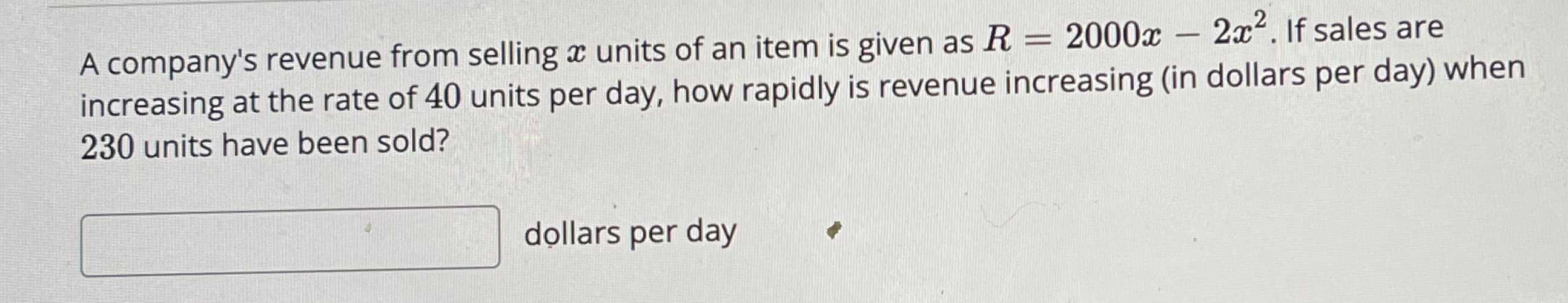### ¿Todavía tienes preguntas de matemáticas?

Pregunte a nuestros tutores expertos
Algebra
PreguntaA company's revenue from selling $$x$$ units of an item is given as $$R = 2000 x - 2 x ^ { 2 }$$ . If sales are increasing at the rate of $$40$$ units per day, how rapidly is revenue increasing (in dollars per day) when $$230$$ units have been sold?